Introduction.
The extra functions are often just a few script lines, so it is not usefull to publish a new version of a plot script with every new line. We just take the standard plot script here.

and indicate where to insert the new fuction. You have to find the equivalent location in your own script.

1. Average multiple spectra lines.
If you have a stable measurement, you could wish to integrate the signal of the source longer than 5 minutes.
Average of a number of 5 minute spectra and save sum file to disk for later use.
Insert after original line 189
#---------------------------------
sumsets=np.zeros((2048)) ;
sumf=3#sum spectrum number from
sumt=23#sum spectrum number to
for sets in range (sumf,sumt):
sumsets=sumsets+outsets[sets]
sumsets=sumsets/(sumt+1-sumf)
np.savetxt(filename+"sum"+str(sumf)+"-"+str(sumt)+".txt",sumsets)# save sum file
#---------------------------------

You can add the average spectrum sum at the bottom of the overview graph.
Insert after original line 192
#---------------------------------
ax.plot(f-Sf,sumsets,linewidth=1)#
#---------------------------------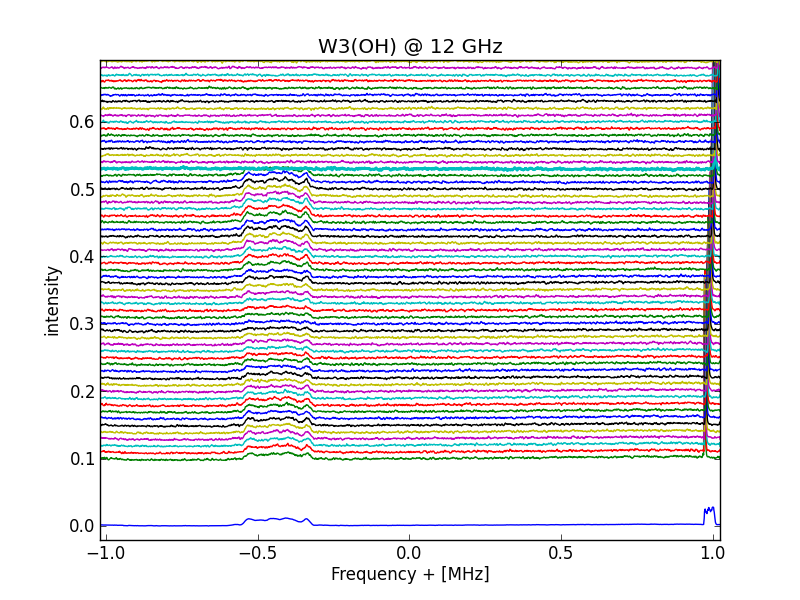Adding multiple 5 minute spectra and plot result

2.Prepare for contour plotting or fits file generation.
To show a contour graph, all the nrad files have to be corrected for the bandpass curve.
Also the spectra base lines have to be normalized; that is; the baseline drift has to be compensated.
If not, you get jumps in the contour lines. See project MK5 to post process the contour graph.
Insert after original line 187
#-------------------------------
offsetcor=1-((data+data+data+data)/4)#line space normalizing
data=data+offsetcor
np.savetxt(filename+str(sets)+".tct",data)#csv preparation. to disable; place # at start of line
#------------------------------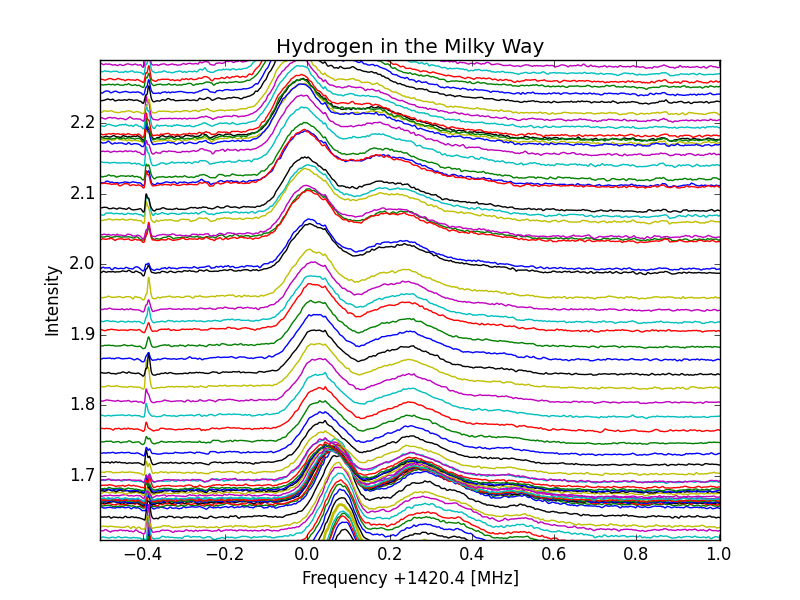No Normalization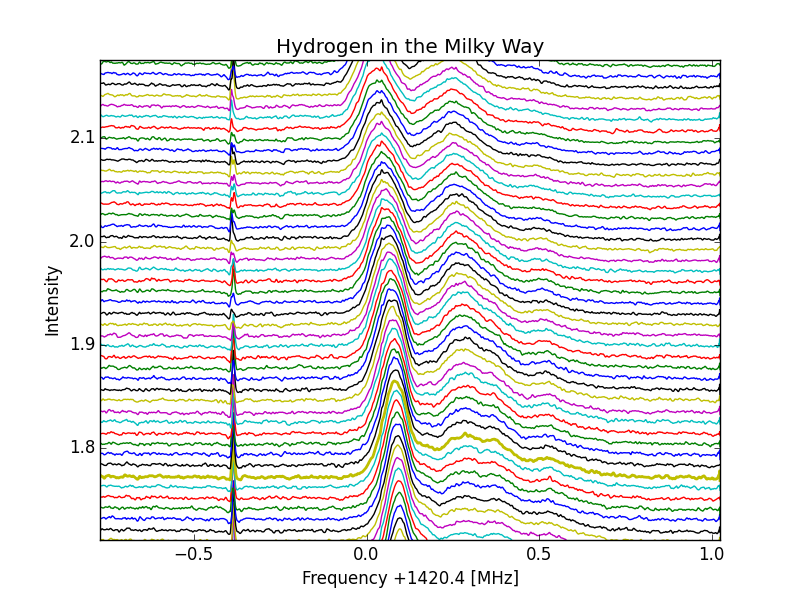With normalization

3. Compensate slanting of the baselines.
Because of temperature drift or meteo differences over a long time, the baselines can start slanting.
This can be compensated . Note that the absolute baseline value is changed by this action, however for frequency comparations this has no effect.
Insert after original line 184
#-------------------------------------
beg=np.mean(data[180:220]#or choose another start value
eind=np.mean(data[1828:1868]#or choose another endvalue
for i in range (0,2048):
data[i]=data[i]+i*(beg-eind)/1648#correct z axis slanting
#-------------------------------------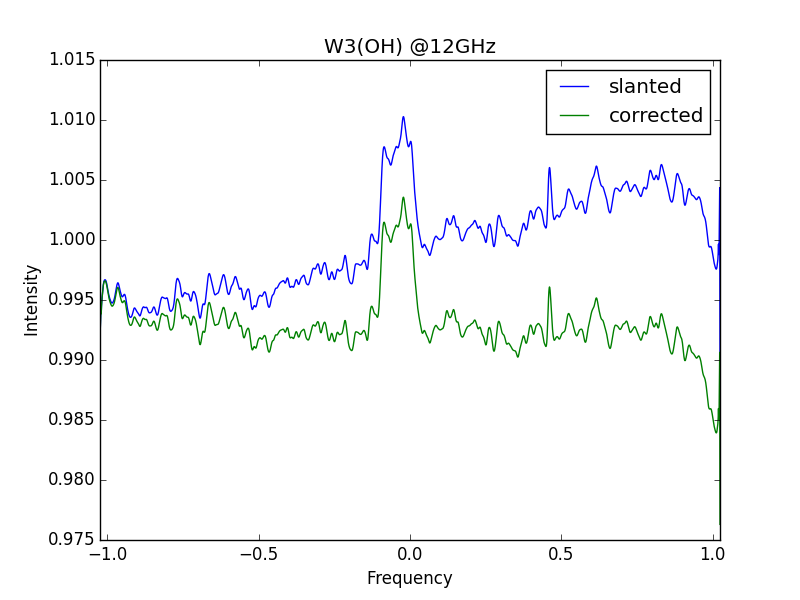W3(OH-12GHz-slanted and corrected

4. Plot all spectra with 2048 BINS as x-axis.
This can be usefull when you want to know in which bin an amplitude effect took place.
Insert after original line 207
#------------------------------------------
fig=mpl.figure(2)
#ax.plot(sumsets,linewidth=1) #average
for i in range (vans,ms+1):
ax.plot((outsets[i]+offsets[i]))
ax.set_title('signal')
ax.set_xlabel('BIN')
ax.set_ylabel('Intensity')
mpl.show()
#------------------------------------------

5. Compensate frequency drift; because of xtal drift.
Use option nr 4 to plot the spectra.
Point with your mouse at a distinct feature of the source curve (first line) and note the bin number=x
Next select the latest line of the source curve for which you want to compensate.
Point again to thesame feature on that last line and note the bin number=y
Count the number of lines between the two selected lines=z.
a=abs(x-y)/z
Remove one of the '#' to select correction of the top to the left or the right.
Insert after original line 164
#------------------------------------------
for set in range (vans,int(sets*a+0.5)) : #shift: a=abs(x-y)/z
for q in range (1,2047):
#             data[q]=dat[q+1] #shift top to left
#             data[2048-q]=data[2048-q-1]#shift top to right
#------------------------------------------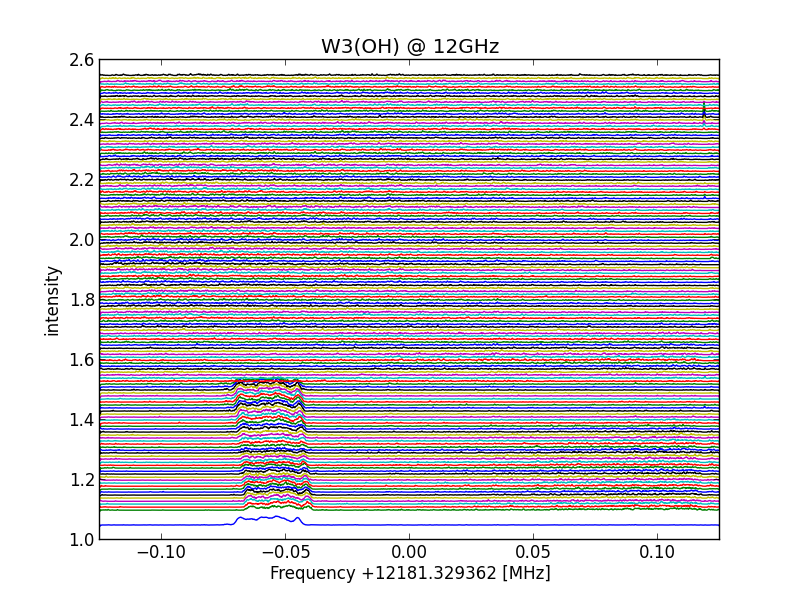temperature frequency drifted linesAll lines shift corrected

6. Reverse spectrum.
There are several ways to do that, but I choose on obvious one.
Replace line 202 with
#------------------------------------------
ax.set_xlim( Sf, -Sf)
#------------------------------------------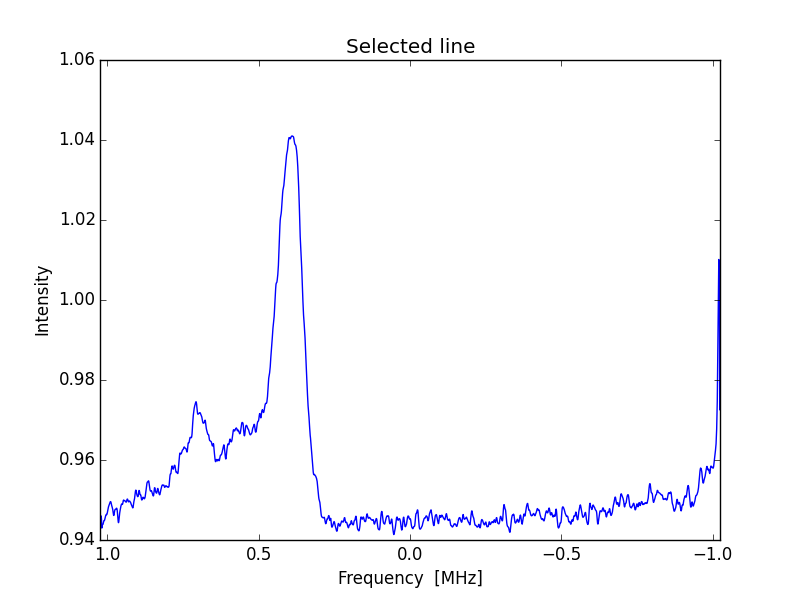X scale corrected curve

7. Adding a second scale; for instance; VLSR.
For neutral hydrogen;
frVLSR=(3e8*(1-(obsfreq-bw/2)/restfreq)/1000-LSR
toVLSR=(3e8*(1-(obsfreq+bw/2)/restfreq)/1000-LSR
restfreq=1420 405 752 Hz
observation frequency =1420 000 000 Hz
bandwidth=2048000 Hz
LSR=Velocity of the Local Standard of Rest of the earth; this includes rearth own rotation and rotation around the sun.
This value can be calculated from;
This value varies between + and - 30 km/s; As a first appoximation; LSR can be set to 0.
Insert after original line 206
#------------------------------------------
ax2 = ax.twiny()
ax2.set_xlim( frVLSR, toVLSR)
ax2.set_xlabel('VLSR [km/s]')
ax2.set_title('Milky Way - 16-6-2014', y=1.07)
#------------------------------------------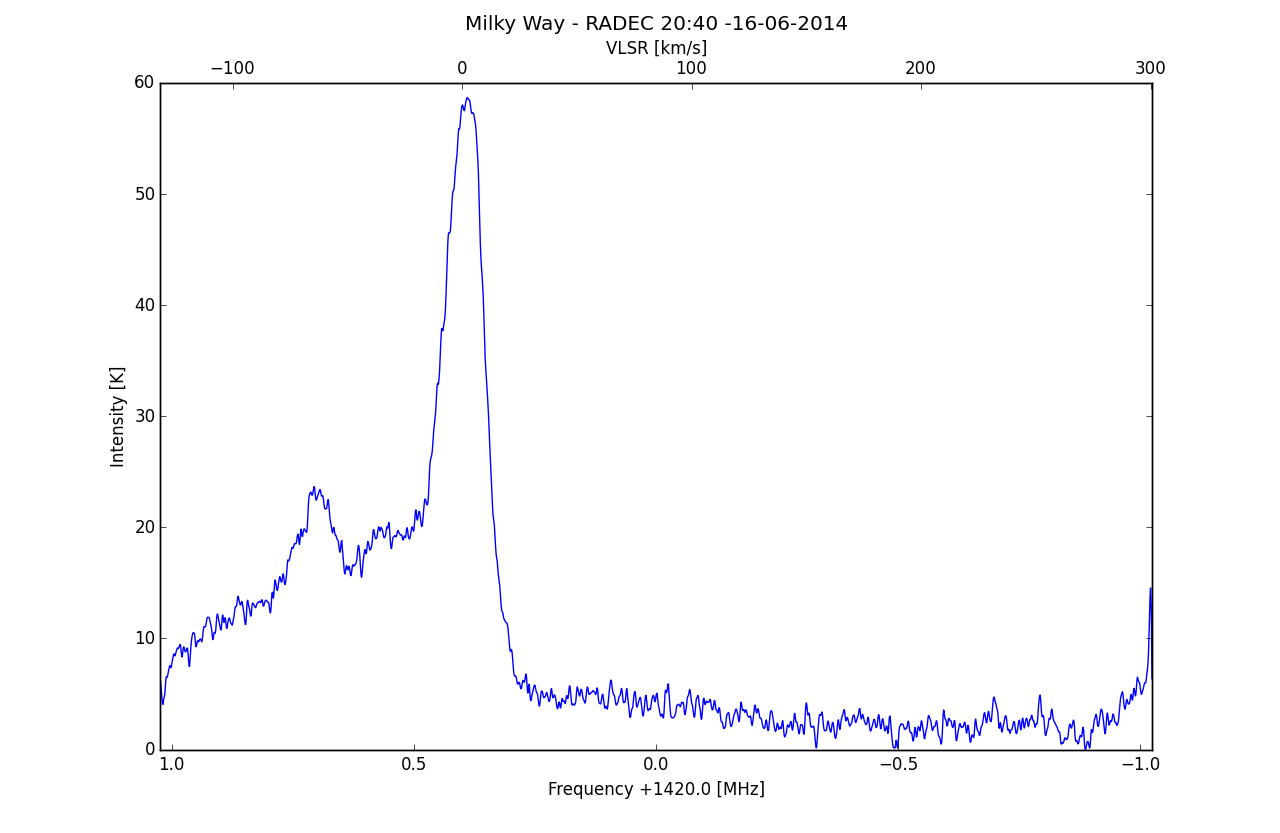8. Change the reference level from 1 to 0.
Replace line 141 with
#------------------------------------------
ax.plot(f-Sf,(raw4)-np.min(raw4), linewidth=1,label='clean')
#------------------------------------------
Or select a 'no signal' region and calculate an average reference value to subtract
#------------------------------------------
ax.plot(f-Sf,(raw4)-np.mean(raw4[300:400]), linewidth=1,label='clean')
#------------------------------------------

9. RFI suppression.
9.1 Valley finding.
In the graph, successive low points are connected, and so pieks are cutt off.
Disadvantage is ; loss of resolution.
The gap to bridge can be set from 1=no gap to 50= large gap, default=10

Insert after original line 125
#------------------------------------------
C=0
D=data#scan start
for i in range (1,2047):
if data[i] <= data[i-1] and data[i]<data[i+1] and (i-C)<10: #dan valley
dalval=data[i] # found valley value
for n in range (1,(i-C)):
data[C+n]=(n*(dalval-D)/(i-C))+data[C]# linear interpolation
D=dalval
C=i
elif data[i] <= data[i-1] and data[i]<data[i+1]:
D=data[i]
C=i
#------------------------------------------

Insert after original line 53 or 164
#------------------------------------------
C=0
D=data#scan start
for i in range (1,2047):
if data[i] <= data[i-1] and data[i]<data[i+1] and (i-C)<10: #dan valley
dalval=data[i] # found valley value
for n in range (1,(i-C)):
data[C+n]=(n*(dalval-D)/(i-C))+data[C]# linear interpolation
D=dalval
C=i
elif data[i] <= data[i-1] and data[i]<data[i+1]:
D=data[i]
C=i
#------------------------------------------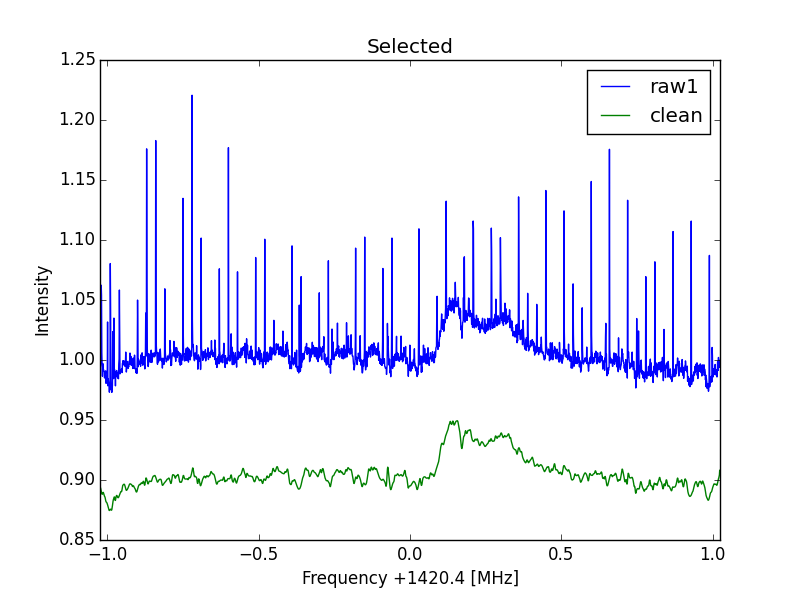RFI suppression

10.Vertical Scale calibration.
Point telescope to strong H1 source like direction RA 20H, DEC=40deg.
Use https://www.astro.uni-bonn.de/hisurvey/profile/ and also fill in the beam of the telescope to get the profile.
Note the peak value of the unibonn curve =UB
Gain correction: GC=UB/RV
Insert after original line 150
#------------------------------------------
fig=mpl.figure(sets)
ax.plot(f-Sf,GC*(raw4-np.min(raw4)), linewidth=1)
ax.set_xlim( -Sf, Sf)
ax.set_title('Selected line')
ax.set_xlabel('Frequency +1420.4 [MHz]') #
ax.set_ylabel('Brightness Temperature [K]')
mpl.show()
#------------------------------------------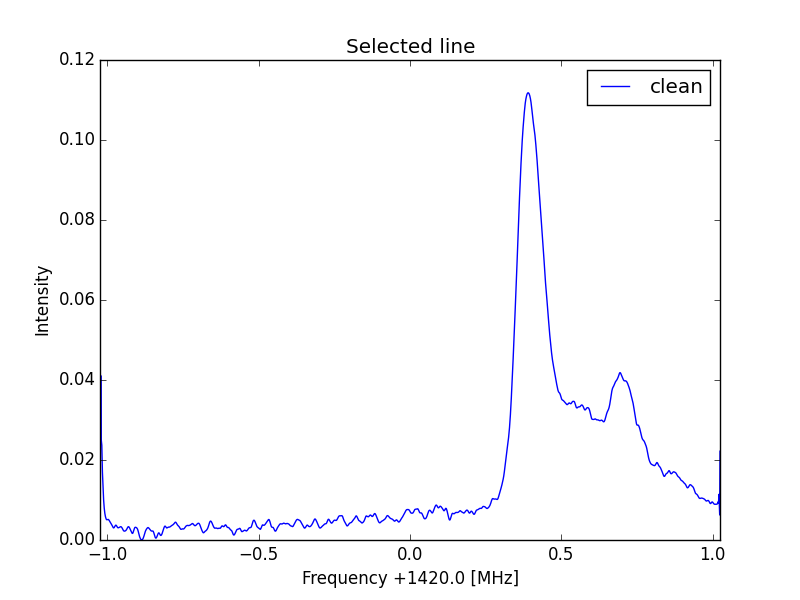Standard curve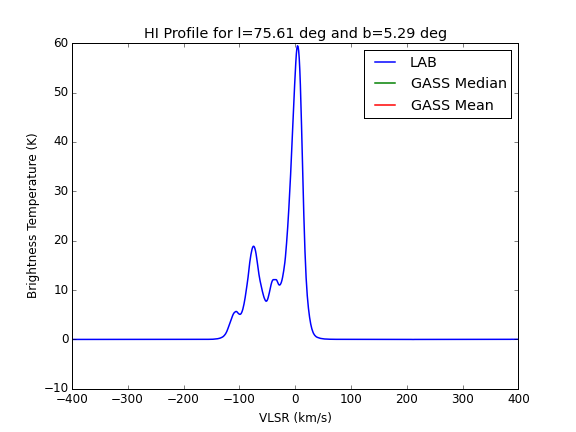Reference curve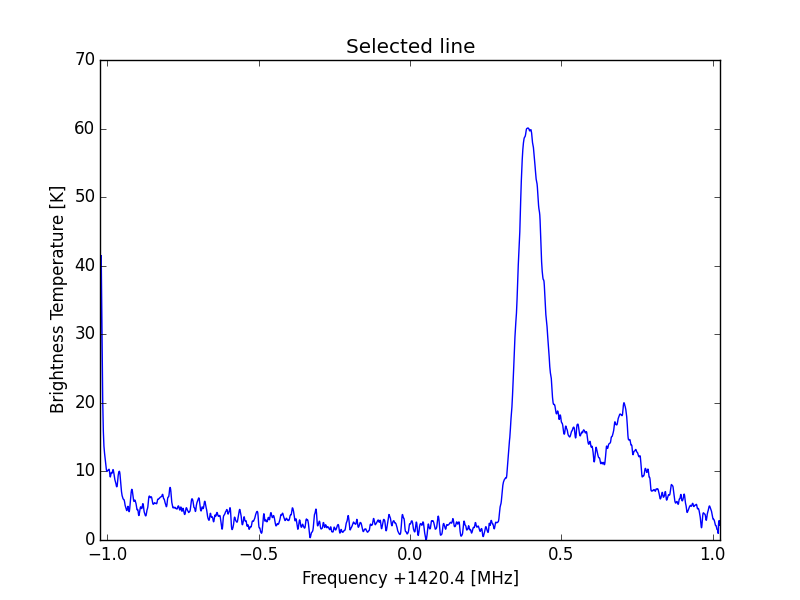Y scale corrected curve

11. Intensity as a function of time.
The spectrum consists of 2048 bins; each bin can be selected as a time signal.
It will be more easy to choose a bin when option 4 is used.
More bins can be chosen also to form an averaging signal.
Insert after original line 207
#------------------------------------------
data=np.zeros((ms+1,2048)) ;
sign1=np.zeros((ms+1,1));#signal 1 of possible 2048 signals
reflow=np.zeros((ms+1,1));#reference
for n in range (0,ms+1):
data[n]=data[n]*bpcorr
for k in range (1407,1408):# select bins of interest
sign1[n]=sign1[n]+data[n,k]
for k in range (500,510): #select quiet part of spectrum (in bins)
reflow[n]=reflow[n]+data[n,k]
sign1=sign1/1
reflow=reflow/10
fig=mpl.figure(2)
ax.plot(516*((sign1-reflow)-np.min(sign1-reflow) ) )#
ax.set_title('Milky Way drift scan')
ax.set_xlabel('*5min from start')
ax.set_ylabel('Brightness Temperature [K]')
mpl.show()
#------------------------------------------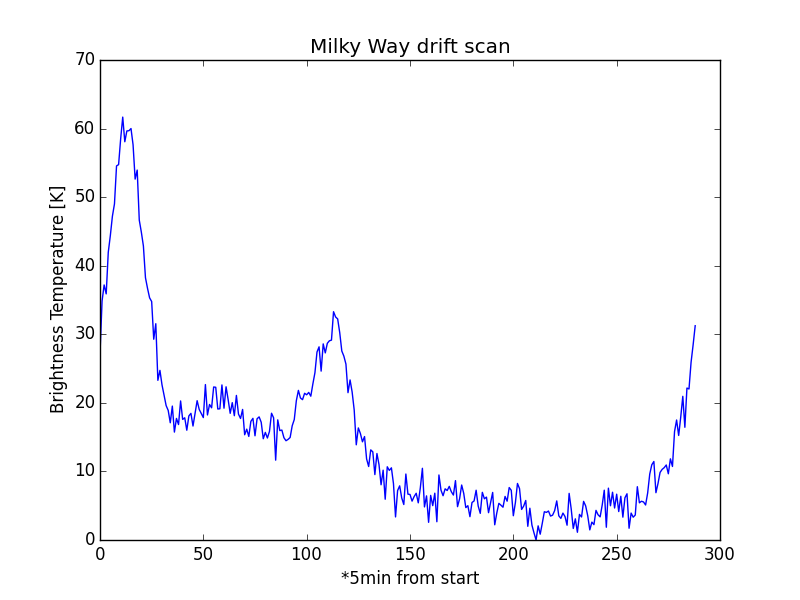Brightness versus Time

Michiel Klaassen 03mrt2020